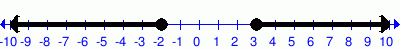# Math Practice Topic: Number Line Inequalities

 Description: Identify graphed number line inequalities. Adaptive Learning Progression: Starts with simple equations, then introduces "and/or" cases. Start using MathScore for free

## Sample Levels (out of 4)

Write the inequality described by the graph using 'x'. Sample answers:   x > 5,   x ≤ -3,   3 < x < 7,   x < -5 or x > 5.

 1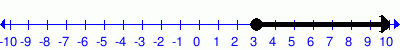2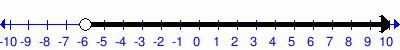Write the inequality described by the graph using 'x'. Sample answers:   x > 5,   x ≤ -3,   3 < x < 7,   x < -5 or x > 5.

 1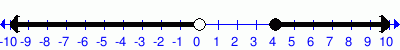2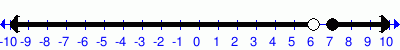34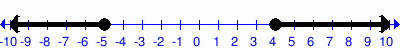5